#Quality Digest

Featured Product
This Week in Quality Digest Live
Statistics Features
Alan Metzel
Introducing the Enhanced Perkin Tracker
Donald J. Wheeler
What you think you know may not be so
Tony Boobier
Why data leaders need to master words as well as statistics
Donald J. Wheeler
What they forgot to tell you in your statistics class
Donald J. Wheeler
How does it compare with a process behavior chart?
Statistics News
New capability delivers deeper productivity insights to help manufacturers meet labor challenges
Day and a half workshop to learn, retain, and transfer GD&T knowledge across an organization
Elsmar Cove is a leading forum for quality and standards compliance
InfinityQS’ quality solutions have helped cold food and beverage manufacturers around the world optimize quality and safety
User friendly graphical user interface makes the R-based statistical engine easily accessible to anyone
Collect measurements, visual defect information, simple Go/No-Go situations from any online device
Good quality is adding an average of 11 percent to organizations’ revenue growth
Ability to subscribe with single-user minimum, floating license, and no long-term commitment
A guide for practitioners and managersBio

Statistics

## The Empirical Rule

### What the average and standard deviation tell you about your histogram

Published: Monday, March 5, 2018 - 12:03

How can we use descriptive statistics to characterize our data? When I was teaching at the University of Tennessee I found a curious statement in a textbook that offered a practical answer to this question. This statement was labeled as “the Empirical Rule,” and it is the subject of what follows.

### The empirical rule

While a statistic may provide a mathematical summary for the data, it has to be understandable before it can truly be said to be descriptive. While the average is easy to understand, most students have trouble understanding the standard deviation statistic. The empirical rule converts the average and standard deviation statistics into comprehensible statements about the data using three intervals centered on the average. The first interval has a radius equal to the standard deviation statistic, the second has a radius equal to twice the standard deviation statistic, and the third has a radius equal to three times the standard deviation statistic.

The three parts of the empirical rule are:
• Part one: Roughly 60 percent to 75 percent of the data will be found within the interval defined by the average plus or minus the standard deviation statistic.
• Part two: Usually 90 percent to 98 percent of the data will be found within the interval defined by the average plus or minus two standard deviations.
• Part three: Approximately 99 percent to 100 percent of the data will be found within the interval defined by the average plus or minus three standard deviations.

But can it really be this simple? Don’t we need to assume that our data are described by some particular probability model before we can compute such specific percentages? In what follows I will attempt to answer these questions by looking at several data sets and then explaining the source of this guide for practice.

We begin with the wire length data. These 100 values have an average of 109.19 and a standard deviation statistic of 2.86. As shown in figure 1, the three intervals of the empirical rule contain, respectively, 69 percent, 95 percent, and 100 percent of the data.Figure 1: The 100 wire length data

Figure 2 shows 200 data from bead-board No. 7. These values have an average of 12.86 and a standard deviation statistic of 3.46. The three intervals of the empirical rule contain, respectively, 66 percent, 97 percent, and 100 percent of the data.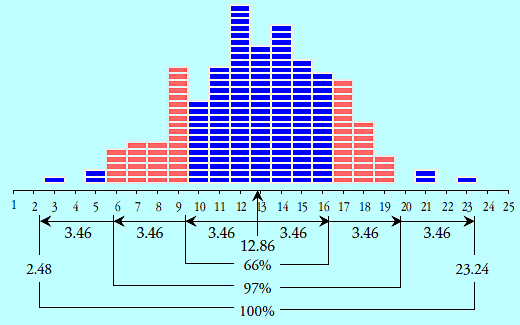Figure 2: 200 data from bead-board No. 7

Figure 3 shows 259 batch weight data. These values have an average of 937.0 kg and a standard deviation statistic of 61.3 kg. The three intervals of the empirical rule contain, respectively, 70.3 percent, 94.6 percent, and 98.8 percent of the data.Figure 3: The 259 batch weight data

Figure 4 shows the 150 camshaft bearing diameter data. These values have an average of 49.81 and a standard deviation statistic of 2.78. The three intervals of the empirical rule contain, respectively, 78.7 percent, 91.3 percent, and 100 percent of the data.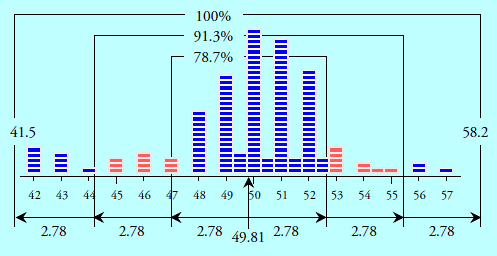Figure 4: The 150 camshaft diameter data

Our fifth example will use the hot-metal delivery times. These 141 values have an average of 59.9 minutes and a standard deviation statistic of 29.7 minutes. As shown in figure 5, the three intervals contain, respectively, 74.5 percent, 92.9 percent, and 98.6 percent of the data.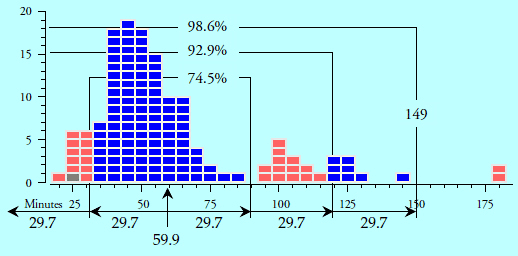Figure 5: The 141 hot-metal delivery-time data

Our sixth example will use the creel yield data. These 68 values have an average of 3492.1 and a standard deviation statistic of 38.3. As shown in figure 6, the three intervals contain, respectively, 59 percent, 98 percent, and 100 percent of the data.Figure 6: The 68 creel yield data

Using the empirical rule, we have made 18 predictions as to where certain percentages of the histograms would be found. Sixteen of the actual percentages fell in the ranges defined by the empirical rule. And depending on how you interpret the word “roughly,” you might argue that the two “misses” with part one were close enough to count.

### Why the empirical rule works

So, the empirical rule uses the descriptive statistics computed from the complete data set to characterize where certain proportions of those data will be found. Once you have computed the average and the global standard deviation statistic for your data you can use the empirical rule to make categorical assertions regarding your histogram.

Moreover, you can make these statements without having to fit a particular probability model to your data. Neither do you need to transform the data to make them “look more normal” in order to make these statements. These proportions are inherent properties of the average and global standard deviation statistic. While some data sets may occasionally fail to satisfy part one, virtually all histograms will satisfy parts two and three of the empirical rule.

Why is this? The answer lies in what these statistics represent. The average is the center of mass for the histogram. It defines the balance point for the data. Most people understand the average. But what does the standard deviation statistic represent?

If we think about the average as the balance point for the histogram, then the global standard deviation statistic is effectively the square root of the rotational inertia of the histogram. What does this mean? Think about having a vertical axis at the average and trying to spin the histogram around this axis. Figure 7 shows two histograms of 68 values. For the same amount of energy the histogram on the right will spin faster than the histogram on the left. This is because the rotational inertia of a histogram will depend upon how the mass of the histogram is spread out. While both histograms have the same mass, the histogram on the left will have the greater rotational inertia. And so rotational inertia characterizes the dispersion of a histogram.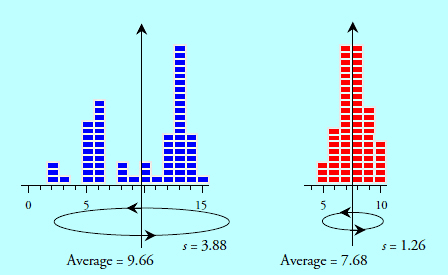Figure 7: The rotational inertia of a histogram

Thus, one of the properties of rotational inertia is that the extreme points possess the greatest amount of rotational inertia. As an example consider our solar system. The sun contains 99.85 percent of the mass in our solar system, leaving only 0.15 percent for the combined mass of all the planets, moons, asteroids, and comets. Yet the four largest planets—Jupiter, Saturn, Uranus, and Neptune—possess 99.8 percent of the rotational inertia of the solar system, and fully 40 percent of the rotational inertia of the solar system belongs to Neptune alone simply because it is so far from the sun.

When we compute a global standard deviation statistic we are essentially computing what physicists call the radius of gyration for the histogram. The radius of gyration is the radial distance from the center of mass where we could concentrate 100 percent of the mass without changing either the center of mass or the rotational inertia. Thus, the radius of gyration for a histogram defines the “balance point” for how the data are spread out on either side of the average. (Technically, it is the root mean square deviation that is the actual radius of gyration, but for convenience we will use the closely related, and more common, standard deviation statistic.) To illustrate how the empirical rule is an natural consequence of using the global standard deviation statistic we shall begin with the simple histogram of figure 8 and modify it. The average value for figure 8 is zero, and the radius of gyration is 1.000. Because there are 100 values in the histogram the standard deviation statistic is: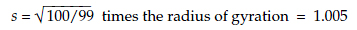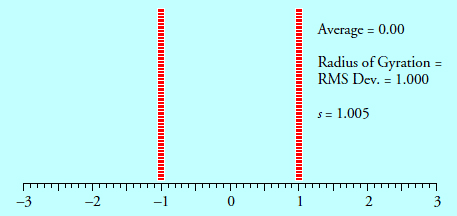Figure 8: A simple histogram

Of course entropy usually prevents us from getting histograms as simple as figure 8, but consider what happens as we move points out of each stack. For simplicity we shall do this symmetrically. If we moved two points out to ±2.58, the standard deviation statistic would increase to s = 1.060. To get the standard deviation back down to the neighborhood of 1.005, we would need to also shift some points into the middle. Four points at zero, six points at ±0.1, and two points at ±0.5 will suffice to bring the standard deviation back down to s = 1.004. Thus, as shown in figure 9, we had to shift 12 points into the middle to compensate for the two points we moved out into the tails.Figure 9: Two points shifted out into the tails

If we now moved an additional six points into the tails by placing two points at ±2.2, two points at ±2.0, and two points at ±1.8, the standard deviation would jump up to 1.092. To compensate for these six points we would have to shift 20 points into the middle by placing two points at ±0.1, eight points at ±0.2, eight points at ±0.3, and two points at ±0.6 to get the standard deviation back down to 1.004 as seen in figure 10.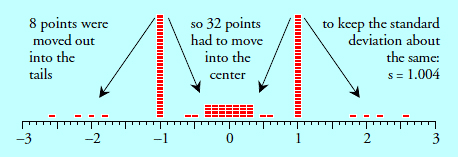Figure 10: Eight points shifted out into the tails

If we now moved an additional 10 points into the tails by placing two points at ±1.7, two points at ±1.6, two points at ±1.5, and four points at ±1.4, the standard deviation would jump up to 1.069. To compensate for these 10 points we would have to shift an additional 18 points into the middle by placing six points at ±0.4, six points at ±0.5, four points at ±0.6, and two points at ±0.7 to get the standard deviation back down to 1.005 as seen in figure 11.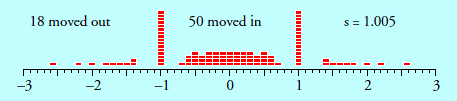Figure 11: 18 points shifted out into the tails

If we now moved an additional 12 points out into the tails by placing four points at ±1.3, four points at ±1.2, and four points at ±1.1, we would have to compensate by shifting 16 points into the middle by placing four points at ±0.7, six points at ±0.8, and six points at ±0.9 to get the standard deviation back down to 1.005 as seen in figure 12. The histogram in figure 12 has a radius of gyration of 1.000, and we find percentages that match the predictions of the empirical rule.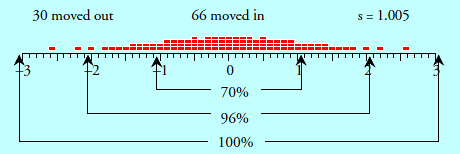Figure 12: 30 points shifted out into the tails

If we think about starting off with all the values at the radius of gyration as in figure 8, any point moved outward will increase the rotational inertia of the histogram. to maintain the same radius of gyration we will have to compensate by moving one or more points inward toward the average. Since rotational inertia is a quadratic operator, we will have to move more points inward than outward. By the time we have moved about one-third of the points outward, we will have had to move the other two-thirds inward to compensate, and this is the basis for the empirical rule. There are limits to how many points we can move outward by various amounts without changing the radius of gyration. And that is why we will always find at least roughly 60 percent within one standard deviation of the average, about 95 percent within two standard deviations of the average, and virtually all of our data within three standard deviations of the average.

### Three-sigma limits

This also explains why we cannot use the global standard deviation statistic to compute limits for a process behavior chart. The global standard deviation does not differentiate between the within-subgroup variation and the between-subgroup variation. It effectively assumes that the histogram is completely homogeneous. Because of this, the global standard deviation statistic provides no leverage to examine the data for homogeneity.

When we want to know if the process producing our data is being operated predictably we have to use the standard statistical yardstick for separating potential signals from probable noise: the within-subgroup variation.

When working with a sequence of individual values the within-subgroup variation is found by using either the average or the median of the successive differences (aka moving ranges). The limits obtained in this way are known as “three-sigma limits” to differentiate them from the “three-standard-deviation limits” computed by part three of the empirical rule. Figures 13 through 18 show the earlier data sets with their three-sigma limits.

By comparing the number of points outside the limits in figures 13 through 18 with the points outside the outer intervals in figures 1 through 6, you can begin to understand the difference between three-standard-deviation limits and three-sigma limits.

Both figure 1 and figure 13 have no points outside the limits. This happens because this process was operated predictably. When a process is operated predictably, the three-standard-deviation limits will be quite similar to the three-sigma limits.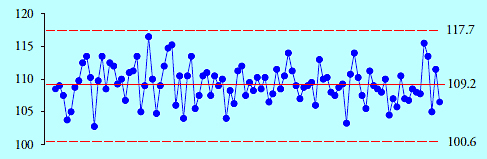Figure 13: X chart for the 100 wire length data

While figure 2 had no points outside the three-standard-deviation limits, figure 14 has 11 out of 200 points (5.5%) outside the three-sigma limits. The data are not homogeneous, so we conclude that the underlying process was operated unpredictably.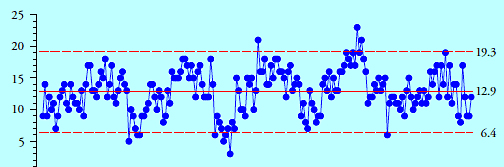Figure 14: X chart for the 200 bead-board data

While figure 3 had three points outside the three-standard-deviation limits, figure 15 has 57 out of 259 points (22%) outside the three-sigma limits. The data are not homogeneous, so we conclude that the underlying process was operated unpredictably.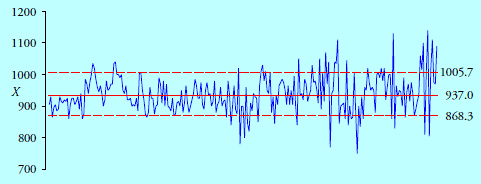Figure 15: X chart for the 259 batch weight data

While figure 4 had no points outside the three-standard-deviation limits, figure 16 has 14 of 150 points (9.3%) outside the three-sigma limits. The data are not homogeneous, so we conclude that the underlying process was operated unpredictably.Figure 16: X chart for the 150 camshaft diameter data

While figure 5 had two points outside the three-standard-deviation limits, figure 17 has seven out of 141 points (5%) outside the three-sigma limits. The data are not homogeneous, so we conclude that the underlying process was operated unpredictably.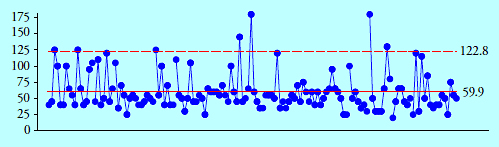Figure 17: X chart for the 141 hot-metal delivery-time data

While figure 6 had no points outside the three-standard-deviation limits, figure 18 has 64 out of 68 points (94%) outside the three-sigma limits. The data are not homogeneous, so we conclude that the underlying process was operated unpredictably.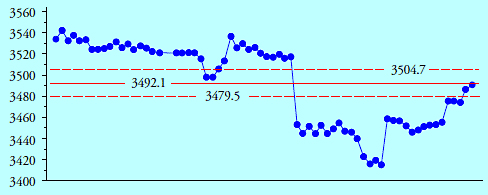Figure 18: X chart for the 68 creel yield data

So, while the empirical rule describes the histogram for both predictable and unpredictable processes, the global standard deviation statistic does not provide the leverage needed to reliably separate predictable processes from unpredictable processes. (Three of the five unpredictable processes above had 100% within the three-standard-deviation interval.) This is the reason why it has always been incorrect to use the global standard deviation statistic when computing limits for a process behavior chart.

Why is this important? While the empirical rule allows us to characterize a histogram, we cannot extrapolate from that histogram to future values until we know that the histogram was generated by a predictable process. Neither can we extrapolate from a histogram to characterize “the product not tested” until we know that the histogram was generated by a predictable process. While some histograms, such as those in figures 4, 5, and 6, can suggest that the underlying process may be unpredictable, no histogram can ever give assurances of having come from a predictable process. Extrapolation requires predictability, and predictability cannot be determined from a histogram.

### Misinterpreting histograms

The elongated tails of figures 3, 4, 5, and 6 illustrate why so many practitioners obsess about their data being non-normal. When a process is operated unpredictably it will move around, and as the process “goes on walkabout,” the histogram will develop extended tails. And these extended tails will tend to mislead those who think that the first step in analysis consists of fitting a probability model to the data.

In practice, when your histogram has an elongated tail, it is much more likely to be due to process changes caused by unpredictable operation than it is likely to be due to the need to fit some exotic probability model to your data.

This is why the idea that you need to prequalify your data before you put them on a process behavior chart is fallacious. You do not have to place your data on a normal probability plot to see if the process behavior chart will work. You do not need to transform the data to make them “look more normal” prior to charting them. And you certainly do not need to fit a probability model to the data prior to using a process behavior chart. If the process is not operated predictably, then the data will not be homogeneous. When the data are not homogeneous the histogram will merely be a pastiche of data coming from a process with multiple-personality disorder. Trying to fit a probability model to nonhomogeneous data is like trying to have a coherent conversation with a schizophrenic who is off his medications.

### Summary

Once you have computed the average and global standard deviation statistic, you have extracted virtually all of the useful information about the histogram that can be obtained from numerical summaries. When used with the empirical rule, these descriptive statistics can be used to summarize the histogram.

Remember that your data are not generated by a probability model. They are generated by some process or operation. And the essential question about this underlying process is whether it has been operated predictably. Unfortunately, the descriptive statistic known as the global standard deviation will not provide any leverage to answer this question. To determine process predictability you will need to use the three-sigma limits that are found on a process behavior chart.

So, learn and use the empirical rule. It is a fundamental property of histograms. When a process is operated predictably, the three-sigma limits and the three-standard-deviation limits will converge as may be seen in figures 1 and 13. When this happens, without regard for the shape of the histogram, you can expect approximately 99 percent to 100 percent of your data to fall within the three-sigma limits of your process behavior chart. Thus, the generality of the empirical rule, plus the fact that three-standard-deviation limits converge to match the three-sigma limits for a predictable process, explain why we do not have to prequalify our data before we place them on a process behavior chart.

Yes, it really is that simple.

### Discuss